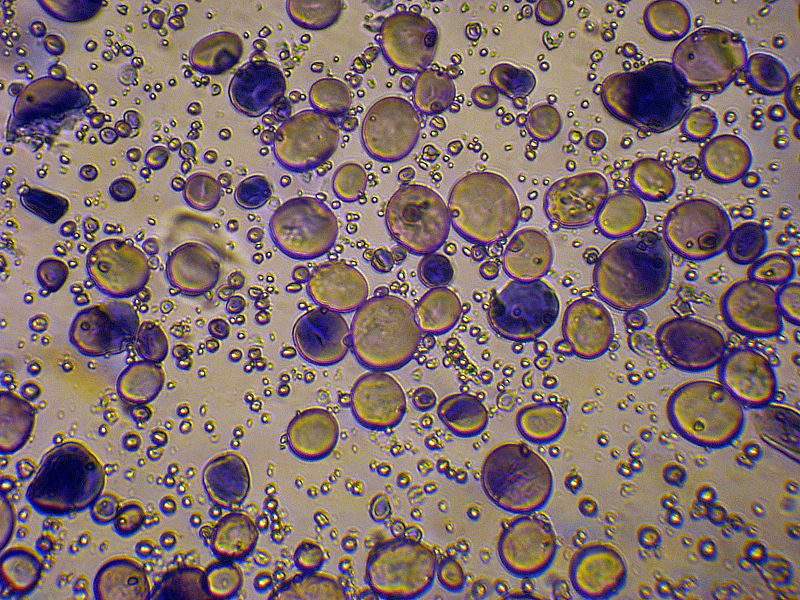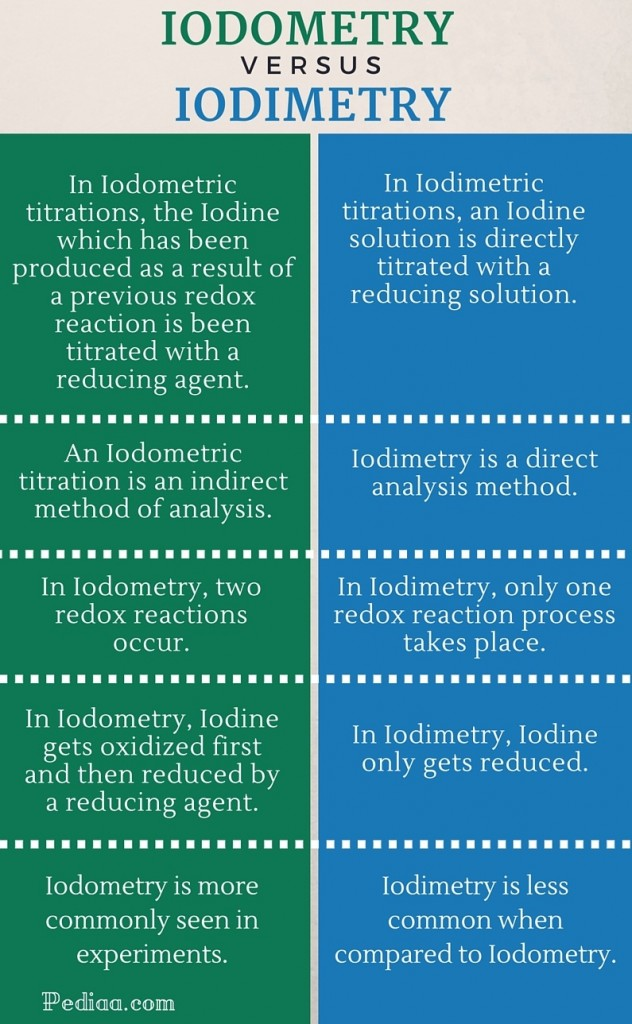# Difference Between Iodometry and Iodimetry

## Main Difference – Iodometry vs Iodimetry

As the names Iodometry and Iodimetry suggest, they relate to a process where Iodine is involved. In fact, both these terms refer to different methods of using Iodine in titrations to determine the concentration of an analyte under investigation. They differ in their approach. Iodometry is an indirect titration method whereas iodimetry is a direct titration method. This is the main difference between Iodometry and Iodimetry.

## What is Iodometry

As mentioned above, Iodometry is an indirect method. In this case, the Iodine, which was produced due to a prior redox reaction, is quantified through a separate titration and the concentration of the analyte that produced the Iodine is  determined. The technique of Iodometry is commonly used in experiments where the amount of oxidizing agents in a water body needs to be quantified.

What happens here is, an excess amount of Iodide solution (typically Potassium Iodide) is mixed with a sample of the water that needs to be tested. Due to the oxidizing agents present in the water body, the Iodide ions get oxidized to Iodine, while the oxidizing agents get reduced. This is the initial redox reaction. Then the produced Iodine is titrated with a reducing agent such as sodium thiosulfate solution. Here, the Iodine reduces to Iodide ions while the thiosulfate ions get oxidized further. This is the second redox reaction and it is the reaction used for the titration. This is performed in the presence of a starch indicator to make it easier to recognize the end point. Iodine forms a deep-blue colour complex with starch and as the Iodine breaks down to Iodide ions, the colour disappears.Starch granules stained with iodine – through microscope

## What is Iodimetry

As mentioned in the definition, this is a direct titration method. The analyte under investigation needs to be the reducing agent. And this is directly titrated with a standard Iodine solution at the presence of a suitable indicator. Therefore, by determining the end point of the reaction, equations can be derived to obtain information of the stoichiometry and other necessary relationships between the reducing agent and Iodine which acts as the oxidizing agent in this case.

Therefore, in this case, only one redox reaction takes place unlike in the case of the Iodometric titration. However, it is more common to use Iodometric methods for analysis rather than Iodimetric methods.## Difference Between Iodometry and Iodimetry

### Definition

In Iodometric titrations, the Iodine which has been produced as a result of a previous redox reaction is been titrated with a reducing agent such as thiosulfate ions.

In Iodimetric titrations, an Iodine solution is directly titrated with a reducing solution.

### Route of Titration

An Iodometric titration is an indirect method of analysis.

Iodimetry is a direct analysis method.

### No. of Redox Reactions

In Iodometry, two redox reactions occur.

In Iodimetry, only one redox reaction process takes place.

### Behaviour of Iodine

In Iodometry, Iodine gets oxidized first and then reduced by a reducing agent.

In Iodimetry, Iodine only gets reduced.

### Usage

Iodometry is more commonly seen in experiments.

Iodimetry is less common when compared to Iodometry.Image Courtesy:

“Wheat starch granules” by Kiselov Yuri – Own work. Licensed under (Public Domain) via Commons

“School level titration demonstration” by UCL – Flickr. (CC BY 2.0) via Commons